• 根号n段归并排序算法时间复杂度分析过程： 1.合并 根号n向下取整 段子数组使用的是自底向上两两归并的策略 2.根号n段归并排序算法时间复杂度的数学推导
• 本文阐述了遗传禁忌搜索算法的混合策略,从理论上对该算法的收敛性进行了证明,对时间复杂度进行了分析。应用马尔科夫链模型证明了遗传禁忌搜索算法是以概率1收敛到全局最优解的,并应用求解随机算法时间复杂度的方法,...
• ## 时间复杂度分析

千次阅读 2020-11-08 16:04:34
时间复杂度分析更多的是对要编写的代码进行一个事前预估分析的一个过程，通过事前大致分析出算法执行的时间和所需要的空间（空间复杂度分析）从而在事前对代码进行调整优化，确保代码的质量。 与事前分析法对用的是...

该节知识点引用机械工业出版社数据结构和算法分析第2章内容

以及极客时间数据结构和算法部分知识点时间复杂度基础分析
算法执行时间分析
时间复杂度分析更多的是对要编写的代码进行一个事前预估分析的一个过程，通过事前大致分析出算法执行的时间和所需要的空间（空间复杂度分析）从而在事前对代码进行调整优化，确保代码的质量。
与事前分析法对用的是事后分析法，事后分析法通过代码在执行后统计代码执行时间和所需要的的资源，但是该方法存在一个不确定性的问题，不同代码在不同配置的计算机上的执行时间可能会不尽相同，而最终得到的结论也是不同的，会存在偏差。
算法的执行效率大致的来说便是算法执行的时间，这里我们通过大O表示法来衡量算法的优劣，O越低的算法执行时间便越低，通常的时间量有O（1），O（n），log(n)，O（n2），O(n3)，等。在代码中一条语句的时间复杂度是O（1），那么是不是可以得到一个结论一个相同的代码执行n此（有限次）那么这条代码的时间复杂度便是O（n）。
O（1）：一个常量级时间复杂度
int x=0;

O（n）：线性级时间复杂度：
int sum=0;
for(int x=0;x<n;x++){
sum +=1;
}

上述代码时间复杂度分析：我定义一个常量级时间复杂度为一个un_time，注意我们讨论的n是有限次的n。
第一行代码：int sum = 0 执行了一次为1个un_time。  第二行代码：for(int x=0;x<n;x++) x=0自增到n（不满足条件退出循环）一共执行n次拿就是n*un_time。  第三行：sum +=1; 注意这里的执行次数是由for循环控制的，那么是不是可以认为我for循环执行了几次第三行就执行了几次，那么是不是就是n*un_time。  现在我们把上述代码的时间复杂度相加便得到整个代码块的时间复杂度（定义为T（n））T（n）=un_time+nun_time+num_time = (2n+1)*un_time
O（n^2）：平方级时间复杂度：
int sum = 0;
for(int x=0;x<n;x++){
for(int y=0;y<n;y++){
sum +=1;
}
}

上述代码时间复杂度分析：
第一行代码：执行了一次花费了一个un_time。  第二行代码：x从0变化到n执行了n次那就是 n*un_time。  第三行代码：这里是重点（和第4行一起分析了）当x=0的时候执行n次，x=1执行n次，x=1时执行n次，x=2时执行n次，x=3时执行n次，那么是不是可以推断出当x=n时执行n*n次，4和4加起来就是(2n^2)*un_time次。  那么总的时间复杂度就是 T（n） = un_time+n*un_time+(2n^2)*un_time = (2n^2+n+1)*un_time
下面对上述代码进行变形：这次我们尝试分析最后一句的语句进行频度
int sum = 0;
for(int x=1;x<=n;x++){
for(int y=1;y<=x;y++){
sum +=1;
}
}

对于上面的代码我们根据O（n2）的时间复杂度分析可以得到一个粗略的值是O（n2）（这里只是一个粗略的估算值，并不是精确值 但是为什么可以取O（n^）后面将会讨论），那么想在 提出的问题是不是计算他的总的时间复杂度而是分析第4行代码的语句执行频度（该行语句的层数是最深的所以执行次数也是最多的，他的执行次数加上2，3行的代码执行次数便是整个代码的精确执行次数）分析：
x=1 --> 1次  x=2 --> 2次  x=3 --> 3次  …  x=n -->n次  可以推出 该语句的执行次数是一个等差数列 a1=1，d=1 通过下述公式计算得语句执行次数为S（n）= (n^2+n)/2;
我们再次对上式进行变形：再次分析sum=sum+1 表达式的语句执行频度
int sum = 0;
for(int x=0;x<=n;x++){
for(int y=0;y<=x;y++){
sum = sum+1;
}
}

我们对上述代码进行分析：
x=0 --> 1  x=1 --> 2  x=2 --> 3  x=3 --> 4  …  x=n --> n+1
我们发现x的变化过程，影响着代码的执行次数 当x=0时代码执行一次，当x=1时代码执行2次那么当x=k（k != n）时代码执行k++次，那么我们将x每次变化的次数相加是不是就能够得到整个执行过程的执行次数，这里我们引用一个数学上的级数公式（下图），我们根据该公式进行求解：通过对T（n） 的计算得到了sum +=1 的代码执行频度是（n+1)*(n+2)/2
O（n^3）：立方级时间复杂度：
int sum = 0;
for(int x=0;x<n;x++){
for(int y=0;x<n;y++){
for(int k=0;k<n;k++){
sum = sum+1;
}
}
}

我们看到上述代码比平方阶多了一层for循环嵌套，通过对O（n2）的计算或许我们能够很快的就计算出T（n）=un_time+n*un_time+n2un_time+n3*un_time+n3un_time 对表达式进行整理：
我们再次对上述代码进行变形：请计算出sum=sum+1的语句执行频度
int sum = 0;
for(int x=1;x<=n;x++){
for(int y=1;y<=x;y++){
for(int k=1;k<=y;k++){
sum = sum+1;
}
}
}

我们发现这个变形代码和上面的代码不同的是他的判断结束条件变了，我们尝试跟踪一下他的执行次数（这里我跟踪的是x的值，执行次数是sum=sum+1）

下面这个图片引用CSDN博客的图片：图片中的i是代码中的x ，j是代码中的yx=1 --> 1  x=2 --> 3  x=3 --> 6  x=4 --> 10  x=i --> i(i+1)/2
根据上述推导可以得到级数公式：对级数公式进行推导计算：我们通过对线性阶，平方阶，立方阶，甚至是4次方阶我们计算其时间复杂度是预测计算取最大值（可以说是最坏情况），在我们精确计算出的数据中我们去其中 最大值最为这个算法的执行时间复杂度例如：三次方阶的标椎代码其时间复杂度我们可以估算为是O（n3），有一个问题我们为什么能够将算法的时间复杂度进行一个估算？在线性阶中一个O（1）相对于一个O（n）那么这个O（1）是不是非常的小，那么这个大O（1）我们是不是可以忽略不计（注意在排序中当一个常量级时间*一个log级时间的时间复杂度是不能进行忽略计算的），那么在O（n3）立方阶中我们是不是也可以取最大值作为整个代码块的时间复杂度。
这里有一点需要注意的是在常量阶中不论赋值语句有多少行，if，switch，等语句有多少都是按照一个O（1）来计算的，只要代码不随n的增长而增长这样的代码便可以记做O（1）
下面我们讨论一下对数（log）阶，对数阶相对于线性阶其算法执行效率，执行时间要更占优（但是个人觉得log阶的时间复杂度也是最难分析的）log阶默认的底都为2如有其它参数作为底请进行特殊标记。
O（logn）：对数阶：
int x=1;
while(x<=n){
x *= 2;
}

从代码中我们可以看出，变量x取值从1开始当x的值大于n时循环结束，那么当x=n 时第三行代码则执行最后一次，通过x的取值变化可以发现他是一个等比数列（2的指数值是该项前面有一个项），那么x的值是不是就是代码的执行次数，2^x = n --> x=logn假设现在对x值得变化进行一个改变令 x *=3 那么执行次数是不是就变成了：‘’那么他的时间复杂度是不是就是 T（n）= log3(n)
我们知道，对数之间是可以互相转换的，log3n 就等于 log32 * log2n，所以 O(log3n) = O(C * log2n)，其中 C=log32 是一个常量。基于我们前面的一个理论：在采用大 O 标记复杂度的时候，可以忽略系数，即 O(Cf(n)) = O(f(n))。所以，O(log2n) 就等于 O(log3n)。因此，在对数阶时间复杂度的表示方法里，我们忽略对数的“底”，统一表示为 O(logn)
2019年考研算法时间复杂度分析题解析:
题目：设 n 是描述问题规模的非负整数，下列程序段的时间复杂度
x=0；
while(n>=(x+1)*(x+1))
)
x=x+l；


解析：代码中使用的是while循环所以我们需要观察x和(x+1)2的变化值和当(x+1)2何时等于n时程序第三行最后执行一次（当(x+1)2>n时程序结束），当找到(x+1)2的值等于n时，该项前面有几项就是程序的执行频度（时间复杂度）：x --> (x+1)^2
0 – > 1  1 --> 4  2 --> 9  3 --> 16  4 --> 25  5 --> 36  …  n --> (n+1)^2
我们运用数学归纳法对数据值进行分析：当(x+1)2=4时程序执行了2此，当(x+1)2=9时程序执行了3次，当(x+1)2=5时程序执行了6次，由此我们是不是暂时得到一个结论当我们(x+1)2的值取根号计算得到代码执行次数，由数学推论法中的特殊性得到普遍性，对(x+1)^进行去根号计算可以得 到代码执行次数，应为(x+1)^2等于n所以可以得出算法执行的时间复杂度为：
加法法则
加法法则：总的时间复杂度等于量级最大那段代码的时间复杂度
//代码片段一
int sum1 = 0;
for(int x=0;x<1000;x++){
sum1 +=1;
}

//代码片段二
int sum2 = 0;
for(int x=0;x<n;x++){
sum2 += 1;
}

//代码片段三
int sum3 = 0;
for(int x=0;x<n;x++){
for(int y=0;y<n;y++){
sum +=1;
}
}

现在我们是不是能够很快的计算出整个代码块的时间复杂度？代码片段一：O（1），代码片段二：O（n），代码片段三：O（n^2），这里有点需要注意的是代码片段一中的代码循环个了1000次是一个常量级执行时间和n没有关系所以时间复杂度是O（1），这里再次强调一下即使代码片段循环执行10000次，20000次他都是一个常量级时间跟n没有关系，n是一个变化的值他可能是100000或者是1000或者是一个无限大的值当n的值越大的时候对算法的执行时间影响就越大，当我们回到时间复杂度分析的概念来说他分析的是算法的执行效率和数据规模之间的关系，其中体现的是数据规模的变化对算法执行效率的影响而常量值是确定的值，所以在计算算法时间复杂度是O（1）常量级时间复杂度可以忽略不计。
现在我们将上述三个代码段的时间复杂度抽象为公式：
我们对上述公式运用加法法则进行计算：
结论：算法总的时间复杂度等于量级最大的那段代码的时间复杂度。
乘法法则
乘法法则：嵌套代码的复杂度等于嵌套内外层代码复杂度的乘积
//代码片段一
int cal(int n){
int sum = 0;
for(int x=0;x<n;x++){
sum += f(x);
}
}

//代码片段二
int f(int n){
int fel = 0;
for(int x=0;x<=n;x++){
fel +=x;
}
return fel;
}

现在我们能够很快分析出代码片段一和代码片段二的算法执行的时间复杂度：T1(n) = O(n) ；T2(n) = O(n) ，现在我们假设T1(n) = G(n)，T2(n) = C(n)，由乘法法则我们可以得到以下的抽象公式：
由上述公式我们可以得到上述算法执行的时间复杂的是O(n^2)，当我们在计算多个函数的嵌套的时间复杂度时需要一层一层 去解析函数，不能以一个常量的时间复杂度去计算。
总结：嵌套代码的复杂度等于嵌套内外层代码复杂度的乘积
内容小结
复杂度也叫渐进复杂度，包括时间复杂度和空间复杂度是用来分析数据规模算法执行效率之间的关系，越高阶的算法时间复杂度越低，算法的时间复杂度可以粗略的表示。我们常用的算法时间复杂度主要有（从左到右排列表示其大小）：
时间复杂度分析图：

图片引用自即极客时间的数据结构和算法之美的课程图片（画得比较好看）摊还分析法和平均分析法
上面几节我们分析了算法执行的时间复杂度，现在我们将要讨论一下算法执行的最好情况，最坏情况了，平均情况，均摊情况。对算法的最好情况，最坏情况的分析主要是对数据规模，以及数据分布特性的分析，应为数据分布的不同导致的算法时间复杂度的不同。
最好情况
我们先看下面这段代码：
//代码片段一
int[] array  = new int[]{1,5,3,7,9,0};
public int search(int key){
int pos = 0;
for(int x=0;x<array.length;x++){
if(array[x] == key){
pos = x;
break;
}
}
return pos;
}

在代码片段一中定义了一个search函数其功能主要是在array数组中查找值为key的数据，当查找到该数据后便返回该数据在array数组中的位置，然后调用break结束循环。
这时我们讨论一个问题：当我们要查找数据1在array中的位置对应的函数参数是search(1)，此时我们先观察数据1在数组中并且在位置0处，当for循环的x值为0的时候就能查找到该元素并且结束循环保存x的值，我们发现此时for循环只执行了一次那么整个代码的时间复杂度是不是就是O(1)。
由上述分析我们可以得到一个结论：最好时间复杂度就是，在理想情况下这段代码执行的时间复杂度（这个理想的时间复杂度会跟随数据的规模和分布特性而发生改变）。
最坏情况
最坏时间复杂度我们借助最好时间复杂度的代码片段一进行分析：
假设现在我们要查找数据0（假设数组中的数据是唯一的没有重复值），对应的代码search(0)，我们观察数据0位于数组的最后一位，当使用for循环查找数据元素便需要遍历n次才能找到数据0的位置，那么可以推出此时的时间复杂度是O(n)。
总结：最坏时间复杂度就是，在最糟糕的情况下，执行这段代码的时间复杂度。
平均情况
我们分析过最好，最坏时间复杂度，但是两者都是处于一种极端情况，发生的概率不大。为了更好的表示程序执行的时间复杂度我们引入平均情况时间复杂度。我希望代码的时间复杂度趋于一种稳定状态不是最好也是最差。
我们要查找数据元素key在数组中的位置，有n+1种情况，该元素在0-n-1种和元素不在数组中，那么我们将所有可能发生的情况除以总情况数是不是就可以得到整个查找过程的时间复杂度？下面我们来分析一下：
在O标记法中我们可以省略掉系数，低阶，产量及时间那么得到的最终结果值是0(n)
但是这个结果并不是准确的，虽然整个查找过程有n+1中情况但是每个情况发生的概率是不一样的，下面我们来分析一下：
查找成功和查找不成功的情况分别是：1/2的1/2；  查找成功是在0-n-1的概率是：1/n；  根据概率乘法得到查找成功的概率是：1/2n，不成功的概率是：1/2 结合所有情况可以得到如下公式：
根据上述公式得到的T(n)的值就是平均情况时间复杂度的值，我使用O表示法省略去系数，低阶，常亮值得到的依然是O(n)。
均摊情况
均摊时间复杂度和平均时间复杂度两者看起来很像但是是两个概念有细微的差别。大部分情况下我们不需要分析程序的最好，最坏，平均时间。而平均时间复杂度只有在特殊的情况下才会使用，均摊时间复杂度是在更特殊的情况下使用，对数据的要求更高。
下面我们分析一段代码来解释这一个过程：

代码引用自极客时间数据结构和算法之美的代码块(这个代码块能够更好的体现均摊时间情况)。

// array表示一个长度为n的数组
// 代码中的array.length就等于n
int[] array = new int[n];
int count = 0;
void insert(int val) {
if (count == array.length) {
int sum = 0;
for (int i = 0; i < array.length; ++i) {
sum = sum + array[i];
}
array = sum;
count = 1;
}
array[count] = val;
++count;
}


上述代码片段执行数据插入数组的一个功能，当数组为空时直接插入数组中，当数组满时先遍历数组计算整个数组中元素的和并将计算的结果存储入数组下标0位中，然后重置count变量，当还有数据插入时循环往复的执行上述步骤。
我们先来分析一下这个代码片段的平均时间复杂度：
这个代码块执行的过程总共有n+1中情况。当数组为空的情况下是我所期望的理想情况即都能够在O(1)的时间复杂度情况下完成插入操作，但是当数组满时这个是最坏情况我们需要花费O(n)的时间复杂度区遍历数组并且对数组进行求和操作，这里有一点需要注意的是每个情况发生的概率都是一样的(我只从插入数据的角度去观察，每次插入的过程都是一样的)，可以得到如下的分析公式：
我观察一下insert插入函数和find查找函数的不同：
find查找函数在极端情况下是0(1)，而insert函数在大多数情况下是O(1)，只有在极少数情况下是O(n)  对于insert插入函数来说O(1)发生的概率是非常有规律的中是在n-1个O(1)后有一个O(n)，然后在有n-1个O(1)，后再是O(n)，这个过程是循环有序有规律的，而find函数充满了不确定性可能是O(1)也可能是O(n)也可能是平均情况。
针对这种情况我们可以使用均摊时间复杂度进行分析，我们观察insert函数每一次操作O(n)操作后面都有n-1个O(1)操作，所有可以吧耗时较多的那次操作均摊到n-1次耗时低的操作上，那么这组连续的操作的均摊时间复杂度就都是O(1)了。
总结一下均摊时间复杂度分析：均摊时间复杂度分析要求对某一数据结构有一连续的操作，而且在该操作中大部分的情况下都是时间复杂度低的操作，只有少部分的操作是耗时操作，而且这些操作之间存在一个连续关系，这个时候我们就能够尝试将这一小部分的耗时操作均摊到耗时低的操作上面。一般情况下均摊时间复杂度就是最好时间复杂度。
展开全文数据结构
• 算法时间复杂度分析 在看一个算法是否优秀时，我们一般都要考虑一个算法的时间复杂度和空间复杂度。现在随着空间越来越大，时间复杂度成了一个算法的重要指标，那么如何估计一个算法的时间复杂度呢？ 时间复杂度...
算法时间复杂度分析

在看一个算法是否优秀时，我们一般都要考虑一个算法的时间复杂度和空间复杂度。现在随着空间越来越大，时间复杂度成了一个算法的重要指标，那么如何估计一个算法的时间复杂度呢？
时间复杂度直观体现
首先看一个时间复杂度不同的两个算法，解决同一个问题，会有多大的区别。 下面两个算法都是用来计算斐波那契数列的，两个算法会有多大的差异。

斐波那契数列（Fibonacci sequence），又称黄金分割数列、因数学家列昂纳多·斐波那契（Leonardoda Fibonacci）以兔子繁殖为例子而引入，故又称为“兔子数列”，指的是这样一个数列：1、1、2、3、5、8、13、21、34、……在数学上，斐波那契数列以如下被以递推的方法定义：F(1)=1，F(2)=1, F(n)=F(n-1)+F(n-2)（n>=3，n∈N*）

第一种：使用递归方式
    /**
* 使用递归方式计算斐波拉契数列
* @param  index 计算的项数
*/
public static long fibonacciUseRecursion(int index){
if(index <= 1){
return index;
}
return fibonacciUseRecursion(index-1) + fibonacciUseRecursion(index-2);
}

第二种：使用非递归方式
    /**
* 不使用递归方式计算斐波拉契数列
* @param index 计算的项数
*/
public static long fibonacciNoUseRecursion(int index){
if (index <= 1){
return index;
}
long first = 0;
long second = 1;
for (int i = 0; i < index - 1;i++){
second = first + second;
first = second - first;
}
return second;
}

对上面两种算法进行简单的运行时间统计，我们使用下面的代码进行简单的测试
public static void main(String[] args) {
// 获取当前时间
long begin = System.currentTimeMillis();
// 计算第50项斐波拉契数列的值
System.out.println(fibonacciUseRecursion(50));
// 计算时间差，算法执行所花的时间
System.out.println("time:" + (System.currentTimeMillis() - begin) / 1000 +"s");

begin = System.currentTimeMillis();
System.out.println(fibonacciNoUseRecursion(50));
System.out.println("time:" + (System.currentTimeMillis() - begin) / 1000 + "s");
}

测试结果如下: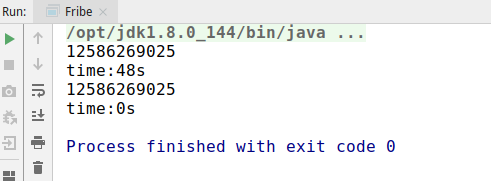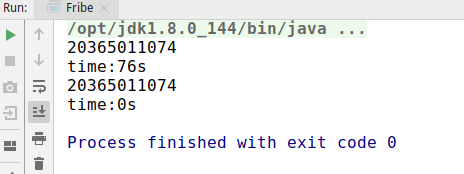可以看到，在计算第50项的时候，第一种递归方式花费了48秒的时间，而第二种不到一秒，虽然这种方式不太科学，但也看出来了两者巨大的差距，并且随着计算的值越大，时间的差异越明显。由此可见，时间复杂度是决定一个算法好坏的重要指标。
如何衡量一个算法的好坏
正确性、可读性、健壮性。 算法必须要保证正确，不正确的算法是没有必要衡量其好坏的；算法也要保证良好的可读性，能够让阅读者明白内在实现与逻辑；健壮性为对不合理输入的反应能力和处理能力，比如非法输入，要有相应的处理，而不应该程序奔溃等。这些都是一个良好的算法必备的条件。时间复杂度 时间复杂度也是一个衡量算法优劣的重要条件，不同的算法的执行时间可能会存在很大的差别。空间复杂度 空间复杂度表示一个算法执行过程中，需要的空间(内存)数量，也是衡量一个算法的重要指标，尤其是在嵌入式等程序中的算法，内存是非常宝贵的，有时候宁愿提高时间复杂度，也要保证不占用太多的空间。
如何计算时间复杂度
第一种：事后统计法
上面我们使用了一种计算执行前后时间差的方式，直观的来看一个算法的复杂度，比较不同算法对同一组输入的执行时间，这种方法也叫作"事后统计法"，但是这种方法也存在一些问题，主要问题有：
执行时间严重依赖于硬件已经运行时各种不确定的环境因素。 比如两个算法在不同的硬件机器上进行测试，硬件不同，运行时间也会存在差异，即使就在一台机器上执行，也会存在运行时机器的CPU、内存使用情况不同等因素。必须要编写相应的测试代码。测试数据的选择难以保证公正性。 比如有两个算法，一个在数据量小的时候占优，一个在大数据量的时候运行较快，这样便难以选择一个公正的测试数据。
第二种：估算代码指令执行次数
那么我们可以使用代码的每个指令的执行次数，可以简单估算代码的执行次数，一般情况下，执行次数少的肯定要比执行次数多的花的时间更少。看如下的示例：
    public static void test1(int n) {
if (n > 10) {
System.out.println("n > 10");
} else if (n > 5) {
System.out.println("n > 5");
} else {
System.out.println("n <= 5");
}

for (int i = 0; i < 4; i++) {
System.out.println("test");
}
}

上面这个方法，我们计算它的执行次数。
最上面的if…else if…else这个判断，判断会执行一次、判断成立的代码会执行一次。下面的for循环，i=0这句赋值会执行一次，i<4这个判断条件会执行4次，i++也会执行4次，循环体(输出语句)也会执行4次。因此，整个方法的执行次数为：1+1+1+4+4+4 = 15次。
    public static void test2(int n) {
for (int i = 0; i < n; i++) {
System.out.println("test");
}
}

上面这个方法，我们计算它的执行次数。
在for循环中，i=0这句赋值会执行一次，i < n执行n次，i++执行n次，循环体执行n次。因此，整个方法的执行次数为：1+n+n+n = 3n+1 次
    public static void test3(int n) {
for (int i = 0; i < n; i++) {
for (int j = 0; j < n; j++) {
System.out.println("test");
}
}
}

上面这个方法，我们计算它的执行次数。
在外层for循环中，i=0这句赋值会执行一次，i < n执行n次，i++执行n次，循环体执行n次。在内层循环中，j=0这句赋值会执行一次，j < n执行n次，j++执行n次，循环体执行n次。因此，整个方法的执行次数为 1+n+n+n*(1+n+n+n)=3n2+3n+1 次
    public static void test4(int n) {
for (int i = 0; i < n; i++) {
for (int j = 0; j < 15; j++) {
System.out.println("test");
}
}
}


上面这个方法，我们计算它的执行次数。
在外层for循环中，i=0这句赋值会执行一次，i < n执行n次，i++执行n次，循环体执行n次。在内层循环中，j=0这句赋值会执行一次，j < 15执行15次，j++执行15次，循环体执行15次。因此，整个方法的执行次数为 1+n+n+n*(1+15+15+15)=48n+1 次
    public static void test5(int n) {
while ((n = n / 2) > 0) {
System.out.println("test");
}
}

上面这个方法，我们计算它的执行次数。
在while循环中，每次对n取一半，相当于对n取以二为底的对数，因此n = n / 2 会执行log2(n)次，判断条件也会执行log2(n)次。在循环体中，这个输出语句也会执行log2(n)次。因此，整个方法的执行次数为 log2(n) + log2(n) + log2(n) = 3log2(n)次
    public static void test6(int n) {
while ((n = n / 5) > 0) {
System.out.println("test");
}
}

上面这个方法，我们计算它的执行次数。
在while循环中，每次对n取五分之一，相当于对n取以五为底的对数，因此n = n / 5 会执行log5(n)次，判断条件也会执行log5(n)次。在循环体中，这个输出语句也会执行log5(n)次。因此，整个方法的执行次数为 log5(n) + log5(n) + log5(n) = 3log5(n)次
    public static void test7(int n) {
for (int i = 1; i < n; i = i * 2) {
for (int j = 0; j < n; j++) {
System.out.println("test");
}
}
}

上面这个方法，我们计算它的执行次数。
在外层for循环中，i= 1执行一遍，每次i翻倍，执行次数为log2(n)，因此i < n会执行log2(n)次，i=i*2会执行log2(n)次，循环体执行log2(n)。在内层for循环中，j=0执行一次，j < n执行n次，j++执行n次，内层循环条件执行n次。因此，整个方法的执行次数为 1+ log2(n) + log2(n) + log2(n)*(1+n+n+n) = 3nlog2(n) + 3log2(n)+1次
    public static void test8(int n) {
int a = 10;
int b = 20;
int c = a + b;
int[] array = new int[n];
for (int i = 0; i < array.length; i++) {
System.out.println(array[i] + c);
}
}

上面这个方法，我们计算它的执行次数。
a=10执行一次，b=20执行一次，c=a+b执行一次，初始化数组执行一次。在for循环中，i=0执行一次，i < 数组长度执行n次，i++执行n次，内层循环条件执行n次。因此，整个方法的执行次数为 1+1+1+1+1+n+n+n =3n +5次。
使用这种方法我们发现计算会特别麻烦，而且不同的时间复杂度表达书也比较复杂，我们也不好比较两个时间复杂度的具体优劣，因此为了更简单、更好的比较不同算法的时间复杂度优劣，提出了一种新的时间 复杂度表示法—大O表示法。
大O表示法

大O表示法：算法的时间复杂度通常用大O符号表述，定义为T[n] = O(f(n))。称函数T(n)以f(n)为界或者称T(n)受限于f(n)。 如果一个问题的规模是n，解这一问题的某一算法所需要的时间为T(n)。T(n)称为这一算法的“时间复杂度”。当输入量n逐渐加大时，时间复杂度的极限情形称为算法的“渐近时间复杂度”。

大O表示法，用来描述复杂度，它表示的是数据规模n对应的复杂度，大O表示法有以下的一些特性：
忽略表达式常数、系数、低阶项。 忽略常数，常数直接为1，比如上面第一个方法的复杂度为15，因此直接取1，其时间复杂度使用大O表示为O(1)。 忽略系数，忽略表达式的系数，比如第二个方法的时间复杂度为3n+1，忽略系数和常数，其时间复杂度为O(n)。 忽略低阶项，比如第三个方法的时间复杂度为3n2+3n+1,忽略低阶项3n，忽略常数1，忽略系数3，则其时间复杂度为O(n2)。对数阶一般忽略底数 对于对数直接的转换，一个对数都可以乘以一个常数项成为一个没有底数的对数，比如 log2n = log29 * log9n，因此可以省略底数，比如上面第五个方法的时间复杂度为log2(n),可以忽略底数2，则其时间负责度为logn。大O表示法仅仅是一种粗略的分析模型，是一种估算，能帮助我们短时间内估算一个算法的时间复杂度。
常见的复杂度
执行次数复杂度非正式术语12O(1)常数阶2n+3O(n)线性阶4n2+zn+2O(n2)平方阶4log2n+21O(logn)对数阶3n+2log3n+15O(nlogn)nlogn阶4n3+3n2+22n+11O(n3)立方阶2nO(2n)指数阶
复杂度的大小关系 O(1) < O(logn) < O(n) < O(nlogn) < O(n2) < O(n3) < O(2n) < O(n!) < O(nn)。
因此上面的十个方法的复杂度如下：
方法名称复杂度大O表式test115O(1)test23n+1O(n)test33n2+3n+1O(n2)test448n+1O(n)test53log2(n)O(logn)test63log5(n)O(logn)test73nlog2(n) + 3log2(n) + 1O(nlogn)test83n+5O(n)
直观对比复杂的的大小
直接看表达式，还是很难判断一些复杂度的大小关系，我们可以借助可视化的一些工具来查看比如https://zh.numberempire.com/graphingcalculator.php，通过该网站我们看到在n变化的情况下，不同表达式的变换情况。
递归斐波拉契数列计算方法的时间复杂度分析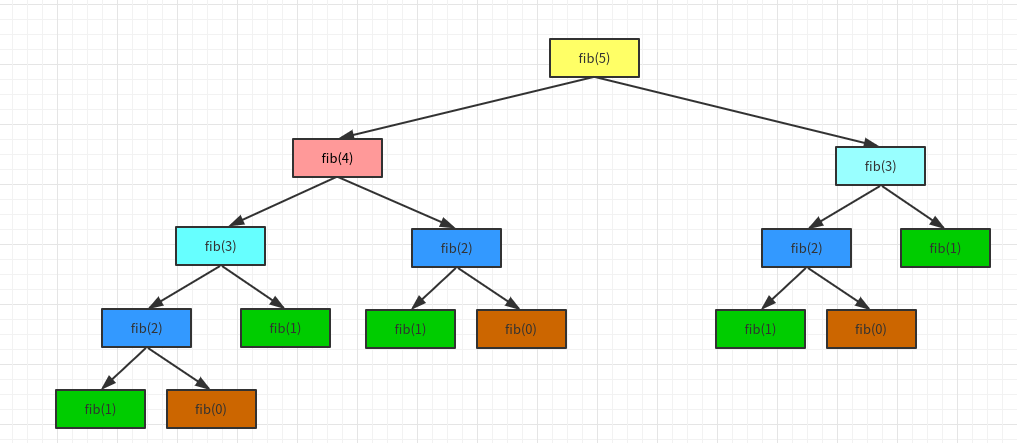第一层计算5，只需要计算1次；第二层计算3和4，2次；计算第3层，4次；计算第4层，8次。所以总共计算1+2+4+8 =15= 25-1 = 1/2 * 22 -1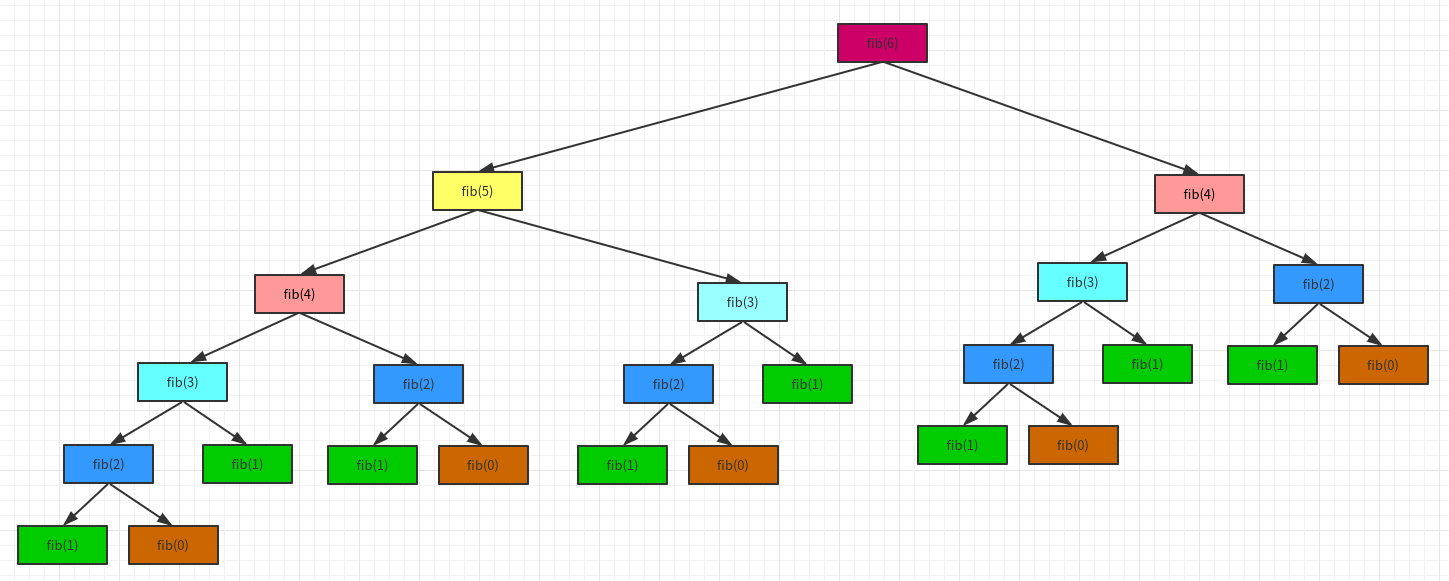第一层计算6，只需要计算1次；第二层计算5和4，2次；计算第3层，4次；计算第4层，8次；第5层，计算10次。所以总共计算1+2+4+8+10 =25 = 25 - 7 = 1/2 * 26 - 7。 所以计算第n项，它的时间复杂度为O(2^n)。 所以最开始的两个算法，第一个的算法复杂度为O(2n),一个为O(n)。 他们的差别有多大？
如果有一台1GHz的普通计算机，运算速度109次每秒（n为64）O(n)大约耗时6.4 ∗ 10-8秒O(2n)大约耗时584.94年有时候算法之间的差距，往往比硬件方面的差距还要大
算法的优化方向
用尽量少的存储空间，即空间复杂度低。用尽量少的执行步骤，即时间复杂度低。一定情况下，时间复杂度和空间复杂度可以互换。
关于复杂度的更多概念
最好、最坏复杂度均摊复杂度复杂度震荡平均复杂度…
总结展开全文算法 java
• 时间复杂度分析的总结 为什么需要复杂度分析？ 刚接触复杂度分析时，我们可能会有些疑惑，直接把写的代码跑一遍，就能得到算法执行的时间和占用的内存大小。为什么还要做时间、空间复杂度分析呢？ 事实上，这种评估...
时间复杂度分析的总结
数据结构和算法本身解决的是“快”和“省”的问题，即如何让代码运行得更快，如何让代码更省存储空间。因此，执行效率是算法一个非常重要的考量指标。
为什么需要复杂度分析？
刚接触复杂度分析时，我们可能会有些疑惑，直接把写的代码跑一遍，就能得到算法执行的时间和占用的内存大小。为什么还要做时间、空间复杂度分析呢？
事实上，这种评估算法执行效率的方法是正确的，我们将其称为事后统计法。但是，这种统计方法有非常大的局限性。
1. 测试结果非常依赖测试环境
测试环境中硬件的不同会对测试结果有很大的影响。比如，同样一段代码，i9 处理器要比 i3 处理器执行的速度快很多。
2. 测试结果受数据规模的影响很大
对同一个排序算法，待排序数据的有序度不一样，排序的执行时间就会有很大的差别。如果数据已经是有序的，那排序算法不需要做任何操作，执行时间就会非常短。如果测试数据规模太小，测试结果可能无法真实地反应算法的性能。
因此，我们需要用到复杂度分析方法，这是一种不用具体的测试数据来测试，就能粗略地估计出算法的执行效率的方法。
大O复杂度表示法
    function fn(n) {
let sum = 0;
let i = 1;
for (; i <= n; i++) {
sum = sum + i;
}
return sum;
}

我们假设每一行代码的运行时间都为1个unit_time，在上述代码中第 2、3 行代码分别需要 1 个 unit_time 的执行时间，第 4、5 行都运行了 n 遍，所以需要 2n*unit_time 的执行时间，所以这段代码总的执行时间T(n)=(2n+2)*unit_time。
再来看下面这段代码
	function fn(n) {
let sum = 0;
let i = 1;
let j = 1;
for (; i <= n; ++i) {
j = 1;
for (; j <= n; ++j) {
sum = sum +  i * j;
}
}
return sum;
}

第 2、3、4 行代码，每行都需要 1 个 unit_time 的执行时间，第 5、6 行代码循环执行了 n 遍，需要2n *unit_time的执行时间，第 7、8 行代码循环执行了n2遍，所以需要 2n2 *unit_time 的执行时间。所以，整段代码总的执行时间 T(n) = (2n2+2n+3)*unit_time。
通过这两段代码执行时间的推导过程，我们可以得到一个非常重要的规律，那就是所有代码的执行时间 T(n) 与每行代码的执行次数 n 成正比。
将这个规律总结为一个公式：T(n)=O(f(n))。
T(n) 表示代码执行的时间；n 表示数据规模的大小；f(n) 表示每行代码执行的次数总和。 O表示代码的执行时间 T(n) 与 f(n) 表达式成正比。因此，第一个例子中的 T(n) = O(2n+2)，第二个例子中的 T(n) = O(2n2+2n+3)。这就是大 O 时间复杂度表示法。
大 O 时间复杂度实际上并不具体表示代码真正的执行时间，而是表示代码执行时间随数据规模增长的变化趋势。
当 n 趋于无穷大时，只需要记录一个最大量级，公式中的低阶、常量、系数三部分并不左右增长趋势，所以都可以忽略。因此，第一个例子中的 T(n) = O(n)；第二个例子中的 T(n) = O(n2)。
时间复杂度分析
如何分析一段代码的时间复杂度？
1. 只关注循环执行次数最多的一段代码
我们在分析一段代码的时间复杂度的时候，只需要关注循环执行次数最多的那一段代码就可以了。这段核心代码执行次数的 n 的量级，就是整段要分析代码的时间复杂度。
    function fn(n) {
let sum = 0;
let i = 1;
for (; i <= n; i++) {
sum = sum + i;
}
return sum;
}

其中第 2、3 行代码都是常量级的执行时间，与 n 的大小无关，所以对于复杂度并没有影响。循环执行次数最多的是第 4、5 行代码，这两行代码被执行了 n 次，所以总的时间复杂度就是 O(n)。
2. 加法法则：总复杂度等于量级最大的那段代码的复杂度
    function fn(n) {
let sum_1 = 0;
let p = 1;
for (; p < 100; ++p) {
sum_1 = sum_1 + p;
}

let sum_2 = 0;
let q = 1;
for (; q < n; ++q) {
sum_2 = sum_2 + q;
}

let sum_3 = 0;
let i = 1;
let j = 1;
for (; i <= n; ++i) {
j = 1;
for (; j <= n; ++j) {
sum_3 = sum_3 +  i * j;
}
}
}

上述代码中第一段代码循环执行了 100 次，所以是一个常量的执行时间，与 n 的规模无关。 第二段代码和第三段代码的时间复杂度分别是 O(n) 和 O(n2)。
综合这三段代码的时间复杂度，我们取其中最大的量级。所以，整段代码的时间复杂度就为 O(n2)。也就是说：总的时间复杂度就等于量级最大的那段代码的时间复杂度。
3. 乘法法则：嵌套代码的复杂度等于嵌套内外代码复杂度的乘积
    function cal(n) {
let ret = 0;
let i = 1;
for (; i < n; ++i) {
ret = ret + f(i);
}
}

function f(n) {
let sum = 0;
let i = 1;
for (; i < n; ++i) {
sum = sum + i;
}
return sum;
}

我们单独看 cal() 函数。假设 f() 只是一个普通的操作，那第 4～6 行的时间复杂度就是，T1(n) = O(n)。但 f() 函数本身不是一个简单的操作，它的时间复杂度是 T2(n) = O(n)，所以，整个 cal() 函数的时间复杂度就是，T(n) = T1(n) * T2(n) = O(n*n) = O(n2)。
几种常见时间复杂度实例分析
1. O(1)
O(1) 只是常量级时间复杂度的一种表示方法，并不是指只执行了一行代码。比如这段代码，即便有 3 行，它的时间复杂度也是 O(1），而不是 O(3)。
 	let i = 8;
let j = 6;
let sum = i + j;

因此，只要代码的执行时间不随 n 的增大而增长，这样代码的时间复杂度都记作 O(1)。
2. O(logn)、O(nlogn)
	 i=1;
while (i <= n)  {
i = i * 2;
}

根据前面说的复杂度分析方法，第三行代码是循环执行次数最多的。所以，我们只要能计算出这行代码被执行了多少次，就能知道整段代码的时间复杂度。
从代码中可以看出，变量 i 的值从 1 开始取，每循环一次就乘以 2。当大于 n 时，循环结束。由此我们可以得到一个式子： 2x=n。求得x=log2n，所以，这段代码的时间复杂度就是 O(log2n)。
修改上述代码
	 i=1;
while (i <= n)  {
i = i * 3;
}

得T(n)=O(log3n)。
实际上，不管是以 2 为底、以 3 为底，还是以 10 为底，我们可以把所有对数阶的时间复杂度都记为 O(logn)。我们知道，对数之间是可以互相转换的，log3n 就等于 log32 * log2n，所以 O(log3n) = O(C * log2n)，其中 C=log32 作为一个系数可以忽略，所以O(log2n) 就等于 O(log3n)。因此，在对数阶时间复杂度的表示方法里，我们忽略对数的“底”，统一表示为 O(logn)。
如果一段代码的时间复杂度是 O(logn)，我们循环执行 n 遍，时间复杂度就是 O(nlogn) 了。O(nlogn) 也是一种非常常见的算法时间复杂度。比如，归并排序、快速排序的时间复杂度都是 O(nlogn)。
3. O(m+n)、O(m*n)
 	function cal(m, n) {
let sum_1 = 0;
let i = 1;
for (; i < m; ++i) {
sum_1 = sum_1 + i;
}

let sum_2 = 0;
let j = 1;
for (; j < n; ++j) {
sum_2 = sum_2 + j;
}

return sum_1 + sum_2;
}

从代码中可以看出，m 和 n 是表示两个数据规模。我们无法事先评估 m 和 n 谁的量级大，所以我们在表示复杂度的时候，就不能简单地利用加法法则，省略掉其中一个。所以，上面代码的时间复杂度就是 O(m+n)。
针对这种情况，原来的加法法则就不正确了，我们需要将加法规则改为：T1(m) + T2(n) = O(f(m) + g(n))。但是乘法法则继续有效：T1(m)*T2(n) = O(f(m) * f(n))。
最好、最坏情况时间复杂度
	// n 表示数组 arr 的长度
function find(arr, n, x) {
let pos = -1;
for (let i = 0; i < n; i++) {
if (arr[i] === x) {
pos = i;
break;
}
}
return pos;
}

上述代码要实现的功能是，在一个无序的数组中，查找变量 x 出现的位置。如果找到了，就退出循环并返回索引值；如果没有找到，就返回 -1。
我们来分析一下这段代码的时间复杂度，因为要查找的变量 x 可能出现在数组的任意位置。如果数组中第一个元素正好是要查找的变量 x，那就不需要继续遍历剩下的 n-1 个数据了，那时间复杂度就是 O(1)。但如果数组中不存在变量 x，那我们就需要把整个数组都遍历一遍，时间复杂度就成了 O(n)。所以，不同的情况下，这段代码的时间复杂度是不一样的。
为了表示代码在不同情况下的不同时间复杂度，就需要引入最好情况时间复杂度和最坏情况时间复杂度两个概念。
最好情况时间复杂度就是，在最理想的情况下，执行这段代码的时间复杂度。就像我们刚刚讲到的，在最理想的情况下，要查找的变量 x 正好是数组的第一个元素，这个时候对应的时间复杂度就是最好情况时间复杂度。
最坏情况时间复杂度就是，在最糟糕的情况下，执行这段代码的时间复杂度。就像刚举的那个例子，如果数组中没有要查找的变量 x，我们需要把整个数组都遍历一遍才行，所以这种最糟糕情况下对应的时间复杂度就是最坏情况时间复杂度。
展开全文算法 数据结构
• 具体点来讲就是我们在实现某一种算法的时候，最终目的就是要求计算机(CPU)在最短的时间内，用最少的内存稳定的输出正确的结果。这一章节主要来理解 “快”，至于“省” 和 “稳”，我会在后续章节进行讲解。 那如何...
金庸武侠中描述一种武功招式的时候，经常会用到 “快、准、狠” 这3个字眼。同样，在计算机中我们衡量一种算法的执行效率的时候也会考量3个方面：“快、省、稳”。
具体点来讲就是我们在实现某一种算法的时候，最终目的就是要求计算机(CPU)在最短的时间内，用最少的内存稳定的输出正确的结果。这一章节主要来理解 “快”，至于“省” 和 “稳”，我会在后续章节进行讲解。
那如何来判断某一段代码运行的是否足够快呢？？有没有一种标准让我们能迅速判断出某A算法比某B算法好呢？？大O复杂度表示法 就是我们要寻找的答案，一般情况下，大O复杂度表示法是用来表示算法性能最常见的正式标记法，接下来就一起来看下这个大O复杂度表示法是个什么东东。
算法时间复杂度的由来
在理解什么是时间复杂度之前，我们需要先了解为什么需要复杂度分析。为了更形象的理解这个问题，我们用一段具体的代码来深入分析。这里有段非常简单的代码，实现了 1,2,3…n 的累加和。
案例1：
// 计算 1, 2, 3…n 的累加和
public class Test {
int cal(int n) {
int sum = 0;
int i = 1;
for (; i <= n; ++i) {
sum = sum + i;
}
return sum;
}
}

如果我们要测试以上面这段代码的执行效率，该如何去测试呢 ？？
起初，我们能想到最简单最直接的方法就是把代码在机器上跑一遍，通过统计、监控，就能得到这段代码所执行的时间和占用的内存大小。既然是这样那为什么还要做时间、空间复杂度分析呢？复杂度分析会比我实实在在跑一遍得到的数据更准确吗？
首先，我可以肯定的说，这种评估算法的执行效率是正确的，并且在某些数据结构和算法的书籍中还专门给这种方法起了个名字—事后统计法。但是这种统计方法有非常大的局限性
1 测试结果极度依赖测试环境 测试环境中的硬件不同会对测试结果有很大的影响。比如案例1的代码分别跑在Intel Core i9 和 Intel Core i3的CPU上运行，很明显i9要比i3快很多。再比如我们在同一时刻在i9上执行运算1024/3，并在i3上运算10+10。正常来讲 10+10 操作比 1024/3 的操作简单很多，但是因为硬件性能的影响，导致i9处理器更快的得出结果。难道我们能说 1024/3 比 10+10 算法性能更高？显然不能！即使是一个初级工程师也应该知道除法运算比加法运算消耗更高！这样就造成我们很难用一个统一的标准去衡量执行时间。
2 测试结果受数据规模的影响很大 后续我们会讲到多种排序算法。对于同一种排序算法，比如快速排序或者插入排序，待排序数据的有序度不一样，排序的执行时间就会有很大差别。另外，对于不同的排序算法，测试数据规模不同也可能导致无法真实的反应排序的性能。比如，我们手上有一组小规模的数据需要做排序操作 int arr[] = {4, 10, 42, 1, 9}; 如果分别使用插入排序和快速排序对 arr 进行排序，我们会发现插入排序比快速排序更快。但是这样不能说明插入排序的性能就比快速排序更高。因为随着arr规模的增长，它的性能会越来越低于快速排序。
综上所述，我们需要一个不用具体的测试数据来测试，用“肉眼”就可以粗略的估计算法的执行效率的方法。而这种方法就是我们今天要讲的 时间复杂度分析方法。

代码复杂度的分析过程
算法的执行时间等于它所有基本操作执行时间之和， 而一条基本操作的执行时间等于它执行的次数和每一次执行的时间的积，如下：
算法的执行时间 = 操作1 + 操作2 + ... + 操作n
操作的执行时间 = 操作执行次数 * 执行一次的时间

我们还是以案例1的代码为例。我们知道java代码经过编译之后，最终会被以字节码的方式由JVM来解释执行相应的指令，那我们可以通过如下命令，分别对Test.java进行编译并查看字节码
javac Test.java    // 编译java代码，生成.class字节码文件
javap -c Test      // 使用javap工具查看字节码指令

执行上述的javap命令之后，得到的字节码指令如下: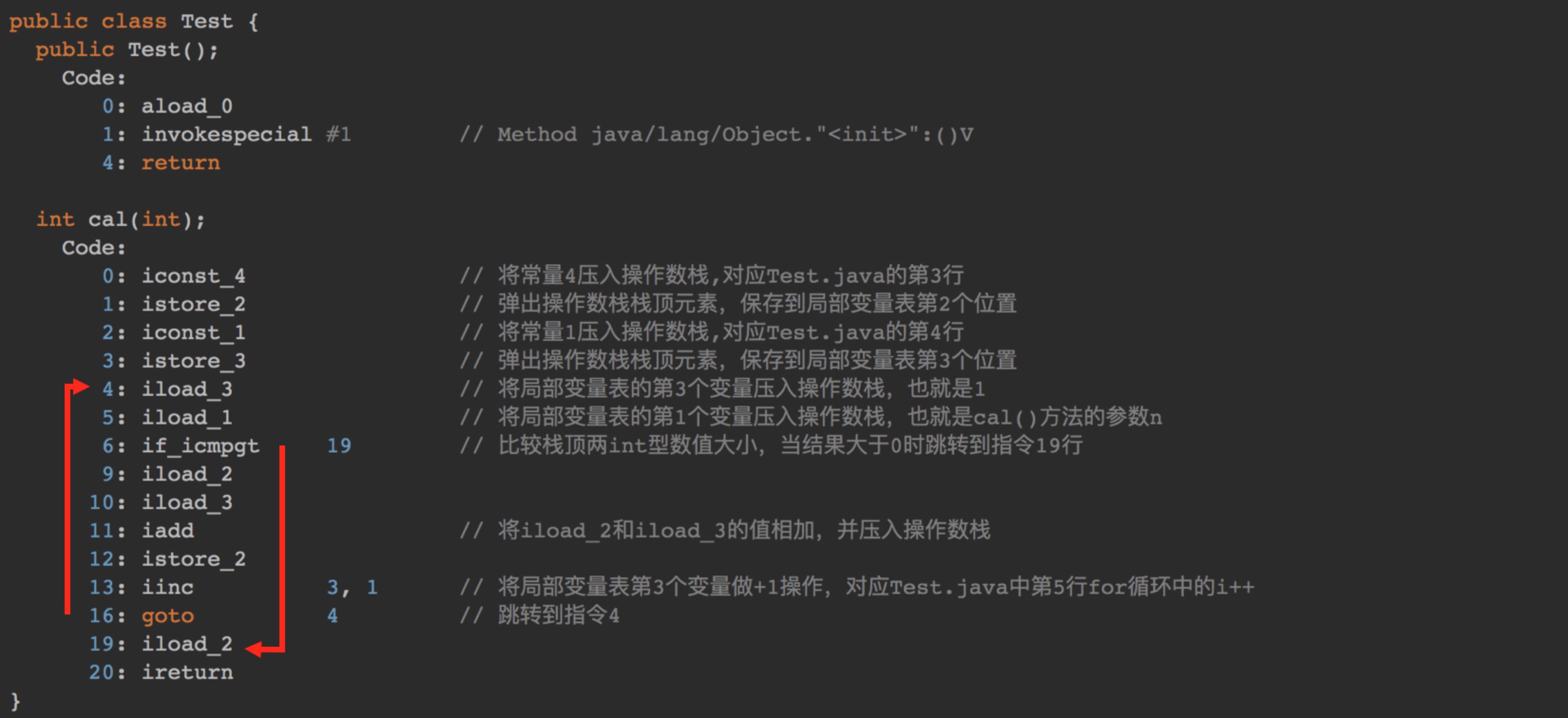可以看出，案例1主要包含的字节码指令就是 iconst_ istore_ iload_ if_icmpgt iadd 等指令，具体每一条指令所代表的意义我已经添加注释(这块内容有点多,但建议耐心仔细阅研读一下，彻底理解每一条指令的意义，也有助于你理解Java代码的运行机制)
然而存在一个问题，不同的编程语言，不同的编译器，或不同的CPU等因素将导致执行一次指令操作的时间各不相同，这样的结果会使算法的比较产生歧义， 于是我们假定所有计算机执行相同的一次指令操作所需时间相同，统一定为 unit_time 。而把算法中基本操作所执行的 执行次数n 作为量度。就是说我们把算法的 运行时间 简单地用基本操作的 运行次数 来代替了, 进而将分析 运行时间 转移到某一行代码的 运行次数

其中unit_time在不同的CPU上可能不一样，比如在i9 Core上有可能是0.01ms，而在i3 Core上可能就是0.05ms。在这个假设的基础上，我们就可以计算出这段代码的总执行时间。

字节码指令是自上而下顺序执行的，所以从指令0开始执行。0-3指令都执行一遍，也就是 **unit_time * 4** 。从指令 4 到指令 19，从图中两个红色箭头也能看出存在循环操作，而
循环的依据就是if_icmpgt指令。
if_icmpgt 指令判断的是操作数栈顶的两个元素的大小，也就是 i 与 n 的大小，因为从指令 4 到指令 19 一共包含10条指令，所以4~19的指令执行次数为：10 * n * unit_time。
综上所述，这段代码总的执行时间就是：

4 * unit_time + 10 * n * unit_time = (10n + 4) * unit_time

按照这个分析思路，我们再来看下面这段代码。
案例2
void cal(int n) {
int sum = 0;
int i = 1;
int j = 1;
for (; i <= n; ++i) {
j = 1;
for (; j <= n; ++j) {
sum = sum +  i * j;
}
}
}

案例2的代码与案例1的唯一区别就是多了一层for循环。经过javac和javap之后的字节码指令如下：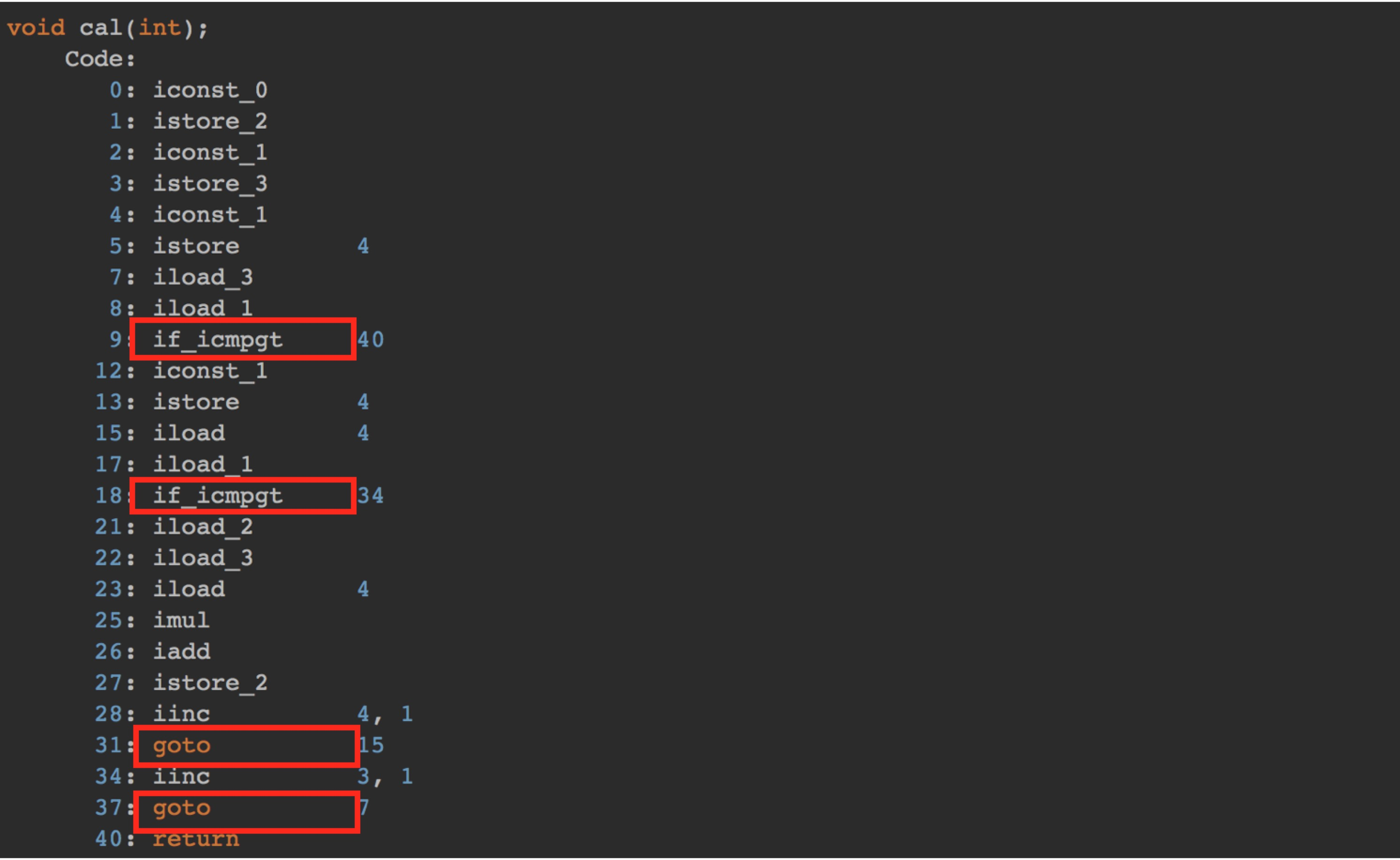分析过程同案例1的一样，具体就不再赘述了。最终分析案例2总的执行时间为：(45n² + 5n + 5) * unit_time
算法时间复杂度表示法
上面我们通过分析字节码指令，计算出案例1和案例2代码片段的具体运行时间，如下：

案例1运行时间 -> (10n + 4) * unit_time案例2运行时间 -> (45n² + 5n + 5) * unit_time

尽管我们不知道 unit_time 的具体值，但是通过对案例1和案例2代码执行时间的推导过程，我们可以得到一个非常重要的规律，那就是 所有代码的执行时间 T(n) 与每行代码的执行次数 n 成正比。
我们可以把这个规律总结成一个公式，注意，大O要登场了
 T(n) = O(f()n)

来解释一下这个公式：T(n)表示代码的执行时间；n表示数据规模的大小；f(n)是一个函数，表示每行代码执行的次数总和。函数f(n)外部的大O表示代码的执行时间 T(n) 与 f(n) 表达式成正比。

注意：大 O 时间复杂度实际上并不具体表示代码真正的执行时间，而是表示代码执行时间随数据规模增长的变化趋势，所以，也叫作渐进时间复杂度(aymptotic time complexity)，简称时间复杂度。或者说它表达的是随着数据规模n的增长，代码执行时间的增长趋势(类似于物理中的加速度)

既然大O表示的是一种增长趋势而不是具体时间，那在我们先前计算出的等式中的 unit_time 也就没什么太大意义了。所以，我们可以将等式中的 unit_time 去掉。

案例1运行时间 -> (10n + 4) *unit_time案例2运行时间 -> (45n² + 5n + 5) *unit_time

最终案例1中的 T(n) = O(10n + 4)，案例2中的 T(n) = O(45n² + 5n + 5)。这就是大 O 时间复杂度表示法。
现在我们了解了大O表示法的演进过程，接下来我们再把视线重新放到案例2的复杂度表示上 T(n) = O(45n² + 5n + 5)，细看之下，此表达式的函数f(n)部分由3部分组成 (45n² 高阶项) 、 (5n 低阶项) 、 (5 常数项)
现在我们假设 n = 3 时

45n² 高阶项 : 45 * 3 * 3 = 405 占总时间的 95.29%5n 低阶项 : 5 * 3 = 155 常数项 : 5

我们已经看到，n²高阶项部分占据了运行时间的大部分比例。
现在，考虑当n = 100时的结果，如下

45n² 高阶项 : 45 * 100 * 100 = 4500 占总时间的 99.98%5n 低阶项 : 5 * 10 = 505 常数项 : 5

可以看到，n²的部分已经占据了几乎整个运行时间，同时其它项的影响力变得更小，试想一下，如果n = 1000, 将占用多少时间！
由此我们可以看出，当我们以增长率的角度去观察 f(n) 函数的时，有几件事就变得很明显：
首先，我们可以忽略常数项，因为随着n的值变得越来越大，常数项最终变得可忽略不计其次，我们可以忽略系数最后，我们只需要考虑高阶项的因子即可，不需要考虑低阶项
综上所述：案例1和案例2的终极大O时间复杂度表达式可以简化为：

案例1: T(n) = O(10n + 4) = O(n) // 时间复杂度为线性级别 案例2: T(n) = O(45n² + 5n + 5) = O(n²) // 时间复杂度为指数级别

时间复杂度简单规则：
前面介绍了大 O 时间复杂度的由来和表示方法。现在我们来看下，当我们拿到一段代码时，如何去分析这一段代码的时间复杂度？
以下是几个比较实用的方法，你可以参考一下：
1 只关注循环执行次数最多的一段代码
我刚才说了，大O这种复杂度表示方法只是表示一种变化趋势。我们通常会忽略掉公式中的常量、低阶、系数，只需要记录一个最大阶的量级就可以了。
所以，我们在分析一个算法、一段代码的时间复杂度的时候，也只关注循环执行次数最多的那一段代码即可。这段代码执行次数的n的量级，就是争端要分析代码的时间复杂度。
为了便于你理解，我还拿前面的例子来说明。
int cal(int n) {
int sum = 0;
int i = 1;
for (; i <= n; ++i) {
sum = sum + i;
}
return sum;
}

其中第 2、3 行代码都是常量级的执行时间，与 n 的大小无关，所以对于复杂度并没有影响。循环执行次数最多的是第 4、5行代码，
所以这块代码要重点分析。前面我们也讲过，这两行代码被执行了 n 次，所以总的时间复杂度就是 O(n)。
2 加法法则：总复杂度登记量级最大的那段代码的复杂度
我这里还有一段代码。你可以先试着分析一下，然后再往下看跟我的分析思路是否一样
int cal(int n) {
int sum_1 = 0;
int p = 1;
for (; p < 100; ++p) {
sum_1 = sum_1 + p;
}
int sum_2 = 0;
int q = 1;
for (; q < n; ++q) {
sum_2 = sum_2 + q;
}
int sum_3 = 0;
int i = 1;
int j = 1;
for (; i <= n; ++i) {
j = 1;
for (; j <= n; ++j) {
sum_3 = sum_3 +  i * j;
}
}
return sum_1 + sum_2 + sum_3;
}

这个代码分为三部分，分别是求 sum_1、sum_2、sum_3。我们可以分别分析每一部分的时间复杂度，然后把它们放到一块儿，再取一个量级最大的作为整段代码的复杂度。
第一段的时间复杂度是多少呢？这段代码循环执行了 100 次，所以是一个常量的执行时间，跟 n 的规模无关。
这里我要再强调一下，即便这段代码循环 10000 次、100000 次，只要是一个已知的数，跟 n 无关，照样也是常量级的执行时间。当 n 无限大的时候，就可以忽略。尽管对代码的执行时间会有很大影响，但是回到时间复杂度的概念来说，它表示的是一个算法执行效率与数据规模增长的变化趋势，所以不管常量的执行时间多大，我们都可以忽略掉。因为它本身对增长趋势并没有影响。
那第二段代码和第三段代码的时间复杂度是多少呢？答案是 O(n) 和 O(n2)，你应该能容易就分析出来，我就不啰嗦了。
综合这三段代码的时间复杂度，我们取其中最大的量级。所以，整段代码的时间复杂度就为 O(n2)。也就是说：总的时间复杂度就等于量级最大的那段代码的时间复杂度。那我们将这个规律抽象成公式就是：

如果 T1(n)=O(f(n))，T2(n)=O(g(n))；那么 T(n) = T1(n)+T2(n) = max(O(f(n)), O(g(n))) = O(max(f(n), g(n)))

3 乘法法则：嵌套代码的复杂度等于嵌套内外代码复杂度的乘积
我刚讲了一个复杂度分析中的加法法则，这儿还有一个乘法法则。当分析一个算法的运行时间时，如果一个任务的执行引起了另一个任务的执行，可以运用此规则。例如，在一个嵌套循环中，外层迭代为T1, 内层迭代为T2, 如果T1 = m, T2 = n, 那么运行结果表示为O(m * n)。
落实到具体的代码上，我们可以把乘法法则看成是嵌套循环，我举个例子给你解释一下
int cal(int n) {
int ret = 0;
int i = 1;
for (; i < n; ++i) {
ret = ret + f(i);
}
}
int f(int n) {
int sum = 0;
int i = 1;
for (; i < n; ++i) {
sum = sum + i;
}
return sum;
}

我们单独看 cal() 函数。假设 f() 只是一个普通的操作，那第 4～6 行的时间复杂度就是，T1(n) = O(n)。但 f() 函数本身不是一个简单的操作，它的时间复杂度是 T2(n) = O(n)，所以，整个 cal() 函数的时间复杂度就是，

T(n) = T1(n) * T2(n) = O(n*n) = O(n²)。

我刚刚讲了三种复杂度的分析技巧。不过，你并不用刻意去记忆。实际上，复杂度分析这个东西关键在于“熟练”。你只要多看案例，多分析，就能做到“无招胜有招”。
总结
这一节我们首先学习了为什么要使用算法复杂度分析，主要是因为外部硬件环境与数据规模不一样，会导致我们的计算结果出现偏差。因此需要一套不依赖具体测试数据的机制来衡量算法的性能。
接下里我们通过分析两个案例代码的粗略执行时间，进而引出了大O复杂度表示法，它是一种正式的表达算法时间复杂度的表示法。需要注意的是大O表达式并不表示某种算法具体的运行时间，而是表示代码执行时间随数据规模增长的变化趋势。
最后我总结了几点分析代码复杂度时的简单规则，或者说是技巧。通过这些技巧有助于我们更快的分析出某一段代码的时间复杂度时多少。

更多文章可以扫描二维码，关注算法公众号展开全文算法复杂度 大O表示法
• 为什么会有时间复杂度分析？ 数据结构和算法解决的就是‘快’和‘省’的问题，即如何让代码跑的更快，更省存储空间。 大 O 复杂度表示法 算法的执行效率，粗略地讲，就是算法代码执行的时间 只关注循环执行次数最多...
• ## DQN算法的时间复杂度分析

千次阅读 多人点赞 2021-05-21 06:43:44
时间复杂度： 设： Initialize replay memory D\mathcal{D}D to capacity NNN (运行消耗t0t_0t0​时间) Initialize action-value function QQQ with random weights (运行消耗t1t_1t1​时间) for episode=1,...DQN 强化学习 复杂度
• 回溯法求解N皇后问题及其时间复杂度分析一、回溯法简介1. 什么是回溯法？2. 回溯法的时间复杂度分析蒙特卡罗方法蒙特卡罗方法在回溯法求解时间复杂度中的应用二、回溯法求解N皇后问题1. 回溯法求解N皇后问题的过程2....算法 c++ N皇后 回溯算法
• ## 归并排序时间复杂度分析

万次阅读 多人点赞 2019-03-19 18:04:57
归并排序时间复杂度分析归并排序工作原理时间复杂度计算如何插入一段漂亮的代码片KaTeX数学公式 归并排序 归并排序也叫（Merge sort）。 工作原理 将给定的数组一份为二 对两部分数组再使用归并排序使其有序 最后再...排序算法 归并 分治算法 算法
• 文章目录Dijkstra算法的思路与关键点Dijkstra算法的时间复杂度 之前一直默认Dijkstra算法时间复杂度为 o(n2)o(n^{2})o(n2)，没有思考过具体的时间复杂度，今天把这个弄清楚。 Dijkstra算法的思路与关键点 思路：...算法 堆栈
• 递归算法中的时间复杂度分析 对于一种算法的时间复杂度分析还是特别重要的，在一些非递归算法中，我们仅仅看运算次数最多的那一行代码可能执行多少次就可以，实际就是看在循环中变量的变化，但是对于递归算法中该...算法 二叉树 数据结构
• 排序(Sorting) 是计算机程序设计中的一种重要操作，它的功能是将一个数据元素（或记录）的...本文主要介绍快速排序算法和归并排序算法的基本概念、原理以及具体的实现方法，并对这两种排序算法的时间复杂度进行分析
• ## 递归算法时间复杂度分析

万次阅读 多人点赞 2018-09-17 16:16:59
递归算法时间复杂度分析 时间复杂度： 一般情况下，算法中基本操作重复的次数就是问题规模n的某个函数f（n），进而分析f（n）随n的变化情况并确定T（n）的数量级。这里用‘o’来表示数量级，给出算法时间复杂度。 T...
• 不同数据范围下，代码时间复杂度和算法该如何选择： n<=30,指数级别，=> dfs+剪枝,状态压缩dp n=100，考虑时间复杂度O（n^3），=> floyd，dp n=1000,考虑时间复杂度O（n^2）或O (n^2longn),=>...
• } } 时间复杂度分析 递归 函数的调用次数可以用二叉树来表示，以n=5为例， 函数的调用次数就等于二叉树的节点个数，而该二叉树的深度m（树中节点的最大层次称为深度，根节点为第一层）为n，如上例深度为5（看最左边...数据结构
• 十大经典排序算法+动图演示+时间复杂度分析 https://www.cnblogs.com/onepixel/articles/7674659.htmljava 算法 排序算法
• 快速排序的时间主要耗费在划分操作上，对长度为n的区间进行划分，共需n-1次关键字的比较，时间复杂度为O(n)。 对n个元素进行快速排序的过程构成一棵递归树，在这样的递归树中，每一层最多对n个元素进行划分，所花...快速排序 排序算法 算法
• 排序算法之 简单选择排序及时间复杂度分析 排序算法之 直接插入排序及时间复杂度分析 排序算法之 希尔排序及时间复杂度分析 排序算法之 快速排序及时间复杂度分析 堆排序 堆的概念： 本质是一种数组对象。特别重要...算法 数据结构 排序算法
• 二分搜索技术的时间复杂度分析 注：我们进行二分搜索的前提是数组a是有序的，否则二分查找结果是不准确的。 解： 令k为查找的次数，s为查找失败时，剩余数组的长度。 当k=1时，s=n/2 k=2时，s=n/22 而当算法
• KMP的时间复杂度分析 假设m为模式串strM的长度，n为待匹配的字符串strN的长度。 O(m+n)=O( [m,2m]+ [n,2n] ) = 计算next数组的时间复杂度+遍历比较的复杂度。 也就是： 计算next数组时的比较次数介于[m,2m]。......